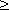# Mathematical induction

Assume it’s necessary to prove a statement ( formula, property etc.), depending on a natural number n . If :

1)  this statement is valid for some natural number n 0 ,

2)  from validity of this statement at n = k its validity follows at n = k + 1 for any kn 0 ,

then this statement is valid for any natural number nn 0 .

E x a m p l e 1.  Prove that  1 + 3 + 5 + ... + ( 2 n – 1 ) = n 2 .

To prove this equality we use the mathematical induction method.

It is obvious that at n = 1 this equality is valid. Assume that it is

valid at some k , i.e. the following equality takes place:

1 + 3 + 5 + ... + ( 2 k – 1 ) = k 2 .

Prove that then it takes place also at k + 1. Consider the correspon-

ding sum at n = k + 1 :

1 + 3 + 5 + ... + ( 2 k – 1 ) + ( 2 k + 1 ) = k 2 + ( 2 k + 1 ) = ( k + 1 ) 2 .

Thus, from the condition that this equality is valid at k it follows,

that it is valid at k + 1 , hence, it is valid at any natural number n ,

which was to be proved.

E x a m p l e 2.  See the solution of the problem 5.047 .

E x a m p l e 3.  See the solution of the problem 5.048 .